### 14.2.2.2 Resolution completeness for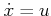Sampling-based notions of completeness can be expressed in terms of reachable sets and the reachability graph. The requirement is to sample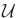in a way that causes the vertices of the reachability graph to eventually become dense in the reachable set, while also making sure that the reachability graph is systematically searched. All of the completeness concepts can be expressed in terms of forward or backward reachability graphs. Only the forward case will be described because the backward case is very similar.

To help bridge the gap with respect to motion planning as covered in Part II, first suppose: 1)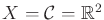, 2) a state is denoted as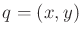, 3)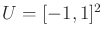, and 4) the state transition equation is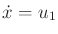and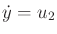. Suppose that the discrete-time model is applied to. Let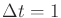and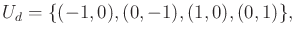(14.9)

which yields the Manhattan motion model from Example 7.4. Staircase paths are produced as was shown in Figure 7.40. In the present setting, these paths are obtained by integrating the action trajectory. From some state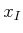, the reachability graph represents the set of all possible staircase paths with unit step size that can be obtained via (14.1).

Suppose that under this model,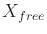is a bounded, open subset of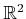. The connection to resolution completeness from Chapter 5 can be expressed clearly in this case. For any fixed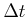, a grid of a certain resolution is implicitly defined via the reachability graph. The task is to find an action sequence that leads to the goal (or a vertex close to it in the reachability graph) while remaining in. Such a sequence can be found by a systematic search, as considered in Section 2.2. If the search is systematic, then it will correctly determine whether the reachability graph encodes a solution. If no solution exists, then the planning algorithm can decreaseby a constant factor (e.g.,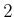), and perform the systematic search again. This process repeats indefinitely until a solution is found. The algorithm runs forever if no solution exists (in practice, of course, one terminates early and gives up). The approach just described is resolution complete in the sense used in Chapter 5, even though all paths are expressed using action sequences.

The connection to ordinary motion planning is clear for this simple model because the action trajectories integrate to produce motions that follow a grid. As the time discretization is improved, the staircase paths can come arbitrarily close to some solution path. Looking at Figure 14.5, it can be seen that as the sampling resolution is improved with respect to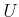and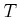, the trajectories obtained via discrete-time approximations converge to any trajectory that can be obtained by integrating some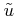. In general, convergence occurs asand the dispersion of the sampling inare driven to zero. This also holds in the same way for the more general case in whichandis any smooth manifold. Imagine placing a grid down onand refining it arbitrarily by reducing.

Steven M LaValle 2020-08-14